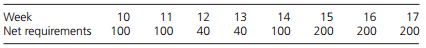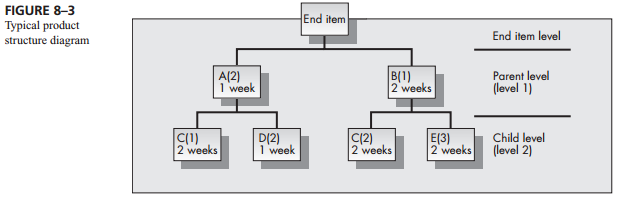Create an Account

Home / Questions / For the example presented in Problem 6 assume that the setup cost for both components A an...

For the example presented in Problem 6 assume that the setup cost for both components A and B is \$100 and that the holding costs are respectively \$0 15 and \$0 25 per component per week Using the

For the example presented in Problem 6, assume that the setup cost for both components A and B is \$100 and that the holding costs are respectively \$0.15 and \$0.25 per component per week. Using the Silver–Meal algorithm, determine the planned order releases for both components A and B and the resulting gross requirements schedules for components C, D, and E.

Problem 6

Consider the product structure diagram given in Figure 8–3. Assume that the MPS for the end item for weeks 10 through 17 isAssume that lot-for-lot scheduling is used throughout. Also assume that there is no entering inventory in period 10 and no scheduled receipts.

a. Determine the planned order release for component A.

b. Determine the planned order release for component B.

c. Determine the planned order release for component C.Jul 28 2020 View more View LessSubscribe To Get Solution